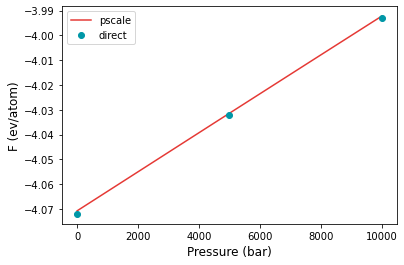# Example 09: Pressure scaling#

In some cases, it is useful to obtain the free energy as a function of the pressure. The calphy mode pscale allows to do this.

The input file is provided in the folder. The pressure scale input file is input2.yaml, in which we calculate the free energy at 1200 K from 0 to 1 GPa. To compare, we do direct free energy calculations at the same temperature and pressures of 0, 0.5 and 1 GPa. The input for this is provided in input1.yaml.

The calculation can be run by:

calphy -i input1.yaml
calphy -i input2.yaml


After the calculations are over, we can read in and analyse the results.

:

import numpy as np
import matplotlib.pyplot as plt

:

p1 = [0, 5000, 10000]
fe1 = [-4.072, -4.032, -3.993]


The above values are taken from the report.yaml files in the corresponding folders.

Note that calphy uses bar as the unit of pressure.

Now read in the pressure scaling results.

:

p2, fe2, ferr2 = np.loadtxt("pscale-fcc-solid-1200-0/pressure_sweep.dat", unpack=True)


Now plot

:

plt.plot(p2, fe2, color="#E53935", label="pscale")
plt.plot(p1, fe1, 'o', color="#0097A7", label="direct")
plt.xlabel("Pressure (bar)", fontsize=12)
plt.ylabel("F (ev/atom)", fontsize=12)
plt.legend()

:

<matplotlib.legend.Legend at 0x7fe37731d0c0>There is excellent agreement between direct calculations and pressure scaling. Now we can also do the calculations for a pressure range of 1-2 GPa. The input is given in input3.yaml and input4.yaml for the pressure scaling and direct calculations respectively.

:

p3 = [10000, 15000, 20000]
fe3 = [-3.994, -3.955, -3.916]

:

p4, fe4, ferr4 = np.loadtxt("pscale-fcc-solid-1200-10000/pressure_sweep.dat", unpack=True)

:

plt.plot(p4, fe4, color="#E53935", label="pscale")
plt.plot(p3, fe3, 'o', color="#0097A7", label="direct")
plt.xlabel("Pressure (bar)", fontsize=12)
plt.ylabel("F (ev/atom)", fontsize=12)
plt.legend()

:

<matplotlib.legend.Legend at 0x7f71512557b0>Once again, for the higher pressure interval, there is excellent agreement between the two methods.